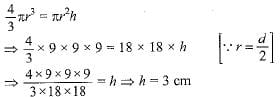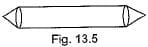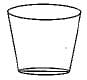RS Aggarwal Test: Surface Area & Volumes

# RS Aggarwal Test: Surface Area & Volumes - Class 10

Test Description

## 10 Questions MCQ Test Mathematics (Maths) Class 10 - RS Aggarwal Test: Surface Area & Volumes

RS Aggarwal Test: Surface Area & Volumes for Class 10 2023 is part of Mathematics (Maths) Class 10 preparation. The RS Aggarwal Test: Surface Area & Volumes questions and answers have been prepared according to the Class 10 exam syllabus.The RS Aggarwal Test: Surface Area & Volumes MCQs are made for Class 10 2023 Exam. Find important definitions, questions, notes, meanings, examples, exercises, MCQs and online tests for RS Aggarwal Test: Surface Area & Volumes below.
Solutions of RS Aggarwal Test: Surface Area & Volumes questions in English are available as part of our Mathematics (Maths) Class 10 for Class 10 & RS Aggarwal Test: Surface Area & Volumes solutions in Hindi for Mathematics (Maths) Class 10 course. Download more important topics, notes, lectures and mock test series for Class 10 Exam by signing up for free. Attempt RS Aggarwal Test: Surface Area & Volumes | 10 questions in 10 minutes | Mock test for Class 10 preparation | Free important questions MCQ to study Mathematics (Maths) Class 10 for Class 10 Exam | Download free PDF with solutions
 1 Crore+ students have signed up on EduRev. Have you?
RS Aggarwal Test: Surface Area & Volumes - Question 1

### If a marble of radius 2.1 cm is put into a cylindrical cup full of water of radius 5 cm and height 6 cm, then how much water flows out of the cylindricall cup?

RS Aggarwal Test: Surface Area & Volumes - Question 2

### The radii of the ends of a frustum of a cone of height h cm are r1 cm and r2 cm . The volume in cm3 of the frustum of the cone is

RS Aggarwal Test: Surface Area & Volumes - Question 3

### A sphere of diameter 18 cm is dropped into a cylindrical vessel of diameter 36 cm, partly filled with water. If the sphere is completely submerged, then the water level rises (in cm) by

Detailed Solution for RS Aggarwal Test: Surface Area & Volumes - Question 3

Volume of sphere
= Volume of water in cylindrical vessel∴ Water level rises by 3 cm

RS Aggarwal Test: Surface Area & Volumes - Question 4

The shape of a gilli, in the gilli-danda game figure is a combination ofRS Aggarwal Test: Surface Area & Volumes - Question 5

A right circular cylinder of radius r cm and height h cm (h > 2r) just encloses a sphere of diameter

RS Aggarwal Test: Surface Area & Volumes - Question 6

A hollow cube of internal edge 22 cm is filled with spherical marbles of diameter 0.5 cm and it is assumed tha 1/8 space of the cube remains unfilled. Then the number of marbles that the cube can accommodate is

RS Aggarwal Test: Surface Area & Volumes - Question 7

During conversion of a solid from one shape to another, the volume of the new shape will

RS Aggarwal Test: Surface Area & Volumes - Question 8

The shape of a glass (tumbler) (see Fig.) is usually in the form ofRS Aggarwal Test: Surface Area & Volumes - Question 9

A mason constructs a wall of dimensions 270 cm x 300 cm x 350 cm with the bricks each of size 22.5 cm x 11.25 cm x 8.75 cm and it is assumed that 1/8 space is covered by the mortar.
Then the number of bricks used to construct the wall is

RS Aggarwal Test: Surface Area & Volumes - Question 10

The radii of the top and bottom of a bucket of slant height 45 cm are 28 cm and 7 cm respectively. The curved surface area of the bucket is

## Mathematics (Maths) Class 10

115 videos|478 docs|129 tests
Information about RS Aggarwal Test: Surface Area & Volumes Page
In this test you can find the Exam questions for RS Aggarwal Test: Surface Area & Volumes solved & explained in the simplest way possible. Besides giving Questions and answers for RS Aggarwal Test: Surface Area & Volumes, EduRev gives you an ample number of Online tests for practice

## Mathematics (Maths) Class 10

115 videos|478 docs|129 tests